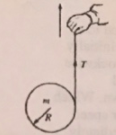# Problem: A solid cylinder of mass m and radius R has a string wound around it. A person holding the string pulls it vertically upward, as shown above, such that the cylinder is suspended in midair for a brief time interval delta-t and its center of mass does not move. The tension in the string is T, and the rotational inertia of the cylinder about its axis is 1/2MR^2The net force on the cylinder during the time interval delta-t is?(A) mg(B) T-mgR(C) mgR-T(D) zero

###### FREE Expert Solution

We're told that the cylinder is in midair.

This implies that the center of mass of the cylinder is at rest.

Let's consider Newton's second law of motion.

95% (221 ratings)###### Problem DetailsA solid cylinder of mass m and radius R has a string wound around it. A person holding the string pulls it vertically upward, as shown above, such that the cylinder is suspended in midair for a brief time interval delta-t and its center of mass does not move. The tension in the string is T, and the rotational inertia of the cylinder about its axis is 1/2MR^2
The net force on the cylinder during the time interval delta-t is?

(A) mg
(B) T-mgR
(C) mgR-T
(D) zero